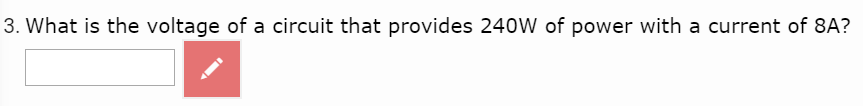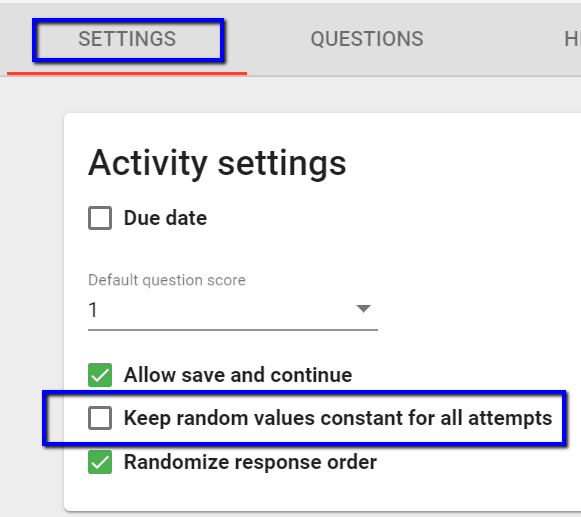# Buzz: Product Feedback

## Numerical range for computation questions

Follow
Feature idea (LEVEL 1)
Science topics often require students to calculate. Currently Buzz does not offer a possibility to specify an accepted range for an answer. I can either provide choices or to make an essay question, both of which have major disadvantages, and the latter requires a manual checking for correctness. A numerical range would require a student to provide an answer free of major errors, would not be suggestive, would not allow to reverse-engineer an answer, and would provide a much better assessment of understanding. In addition, it would be very valuable, if we could specify the number of significant digits in an answer. A screencast with a demo https://drive.google.com/open?id=1YEdntzIahtvVVM4P9Lpey3optVOuO4zm

Sort by

You can *mostly* do what you are asking. It sounds like you want to use the variables. You can use the values of the variables to calculate your answer. The significant digits feature is more of an issue. There is a check box in Buzz that says significant figures, but the chemistry teachers at my school (I teach math) have indicated that it doesn't actually calculate significant digits the way it should. Also, whenever Buzz looks for a numerical answer the default is to count anything within 1% as correct. You can, however change that percent error if you wish.

I can (and have) spend hours going through all of the ins and outs of variables. Since that isn't really appropriate here, I'll suffice to post an example or two using your power and current question.

Hopefully, Brian or someone from Agilix will follow up with a link to a help document explaining variables more fully.

1

Ok, here we go. I'm going to throw a lot at you here. First, I'm giving you the code for two multiple choice questions. I suggest you copy all of the code for both questions. Then make an assessment to test it out. When you get to the question tab click the edit all button <> and paste the code. Then you should be able to preview it. Following the code is a screenshot of what the questions look like for the students. Once you have my code that works you can manipulate it to figure out how it works and alter it to suit your needs.

First, I created a variable named W. I defined it as a number from 160 to 320 counting by 40. So 160, 200, 240, 280, etc. Then I created a variable named A. A is specifically the numbers 2, 4, 5, 8, 10 because I know they will divide into the watts without a decimal. To call the variable into the question you put dollar signs around the name. Notice the extra W and A are the units. For the multiple choice answers I used the evaluate function to calculate the answers. I tried to choose what I thought would be common student errors such as multiplying or dividing wrong. I couldn't think of a fourth so I just added 10 to one of the other answers. Because the question is going to display the results of the evaluation I need a \$ in front and the ,# at the end. After the , is where you tell it the format of the answer. The # represents a blank space to be filled by the answer. So ,# represents an integer answer. Because I know that the reciprocal is going to be a decimal, not an integer I used the format ,0.## which will display a leading 0 then the decimal point followed by the answer out to the hundredths place.

Type: MC
Var: W = 160..320,40
Var: A = 2,4,5,8,10
1) What is the voltage of a circuit that provides \$W\$W of power with a current of \$A\$A?
*a. \$eval(\$W\$/\$A\$,#)V
b. \$eval(\$W\$*\$A\$,#)V
c. \$eval(\$A\$*\$W\$+10,#)V
d. \$eval(\$A\$/\$W\$,0.##)V

Type: MC
Var: W = 160..320,40
Var: A = 2,4,5,8,10
2) What is the voltage of a circuit that provides \$W\$W of power with a current of \$A\$A?
*a. \$eval(\$W\$/\$A\$,#)V
b. \$eval(\$W\$/\$A\$-10,#)V
c. \$eval(\$W\$/\$A\$+10,#)V
d. \$eval(\$W\$/\$A\$+20,#)V0

Here is an example of a fill-in question. Again, click edit <> all in the question editor and paste this code at the end.

In the Type: it says Match. In the visual editor you would have selected answer type Math - exact. I could have used the answer type number instead, but then the students wouldn't be able to enter the V for the units. You can see the setup for the question is the same. The calculation for the answer is the same. Because the answer calculated by the eval function is not going to be displayed for anyone to see, I don't need the \$ in front, nor do I need the ,# display format. As an added feature, I put in two alternate answers. The first one is just the numeric answer without the units. The second is the answer but without the units capitalized. Each of those would grant the student half credit.

Type: F, Match
Score: Partial
Var: W = 160..320,40
Var: A = 2,4,5,8,10
3) What is the voltage of a circuit that provides \$W\$W of power with a current of \$A\$A?
__________
a. eval(\$W\$/\$A\$)V
[0.5] eval(\$W\$/\$A\$)
[0.5] eval(\$W\$/\$A\$)v0

One last thing. If you are making an assignment using the activity type Practice Questions (Formerly Homework) you should go to the settings tab and uncheck the option to keep random values constant for all attempts. For some reason this is checked by default.

Practice Questions let the students redo questions over and over for mastery. If you leave the box checked, the student will get the same numbers every time they retry the question. If you uncheck it the variables will pull new numbers with every retry.0

The variables provide some help, but it is not what I'm after. I would like to be able to specify a numerical range for acceptable answer, with this range hidden from student. A student would have to perform calculation/s and write a number, which cannot be too far from a true number to be correct. This is different from providing choices for a student to select from.

0